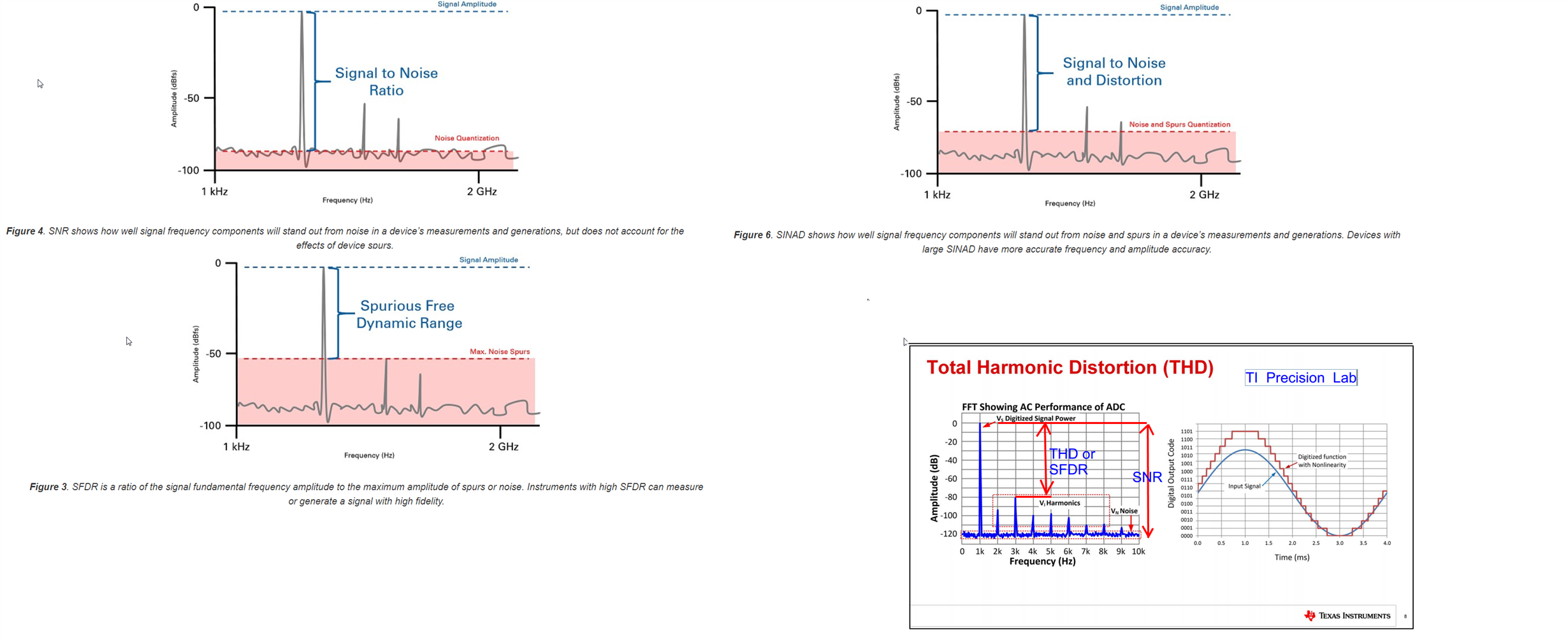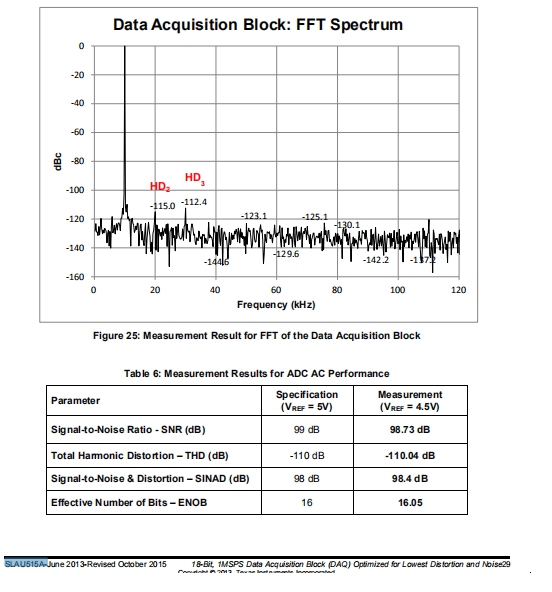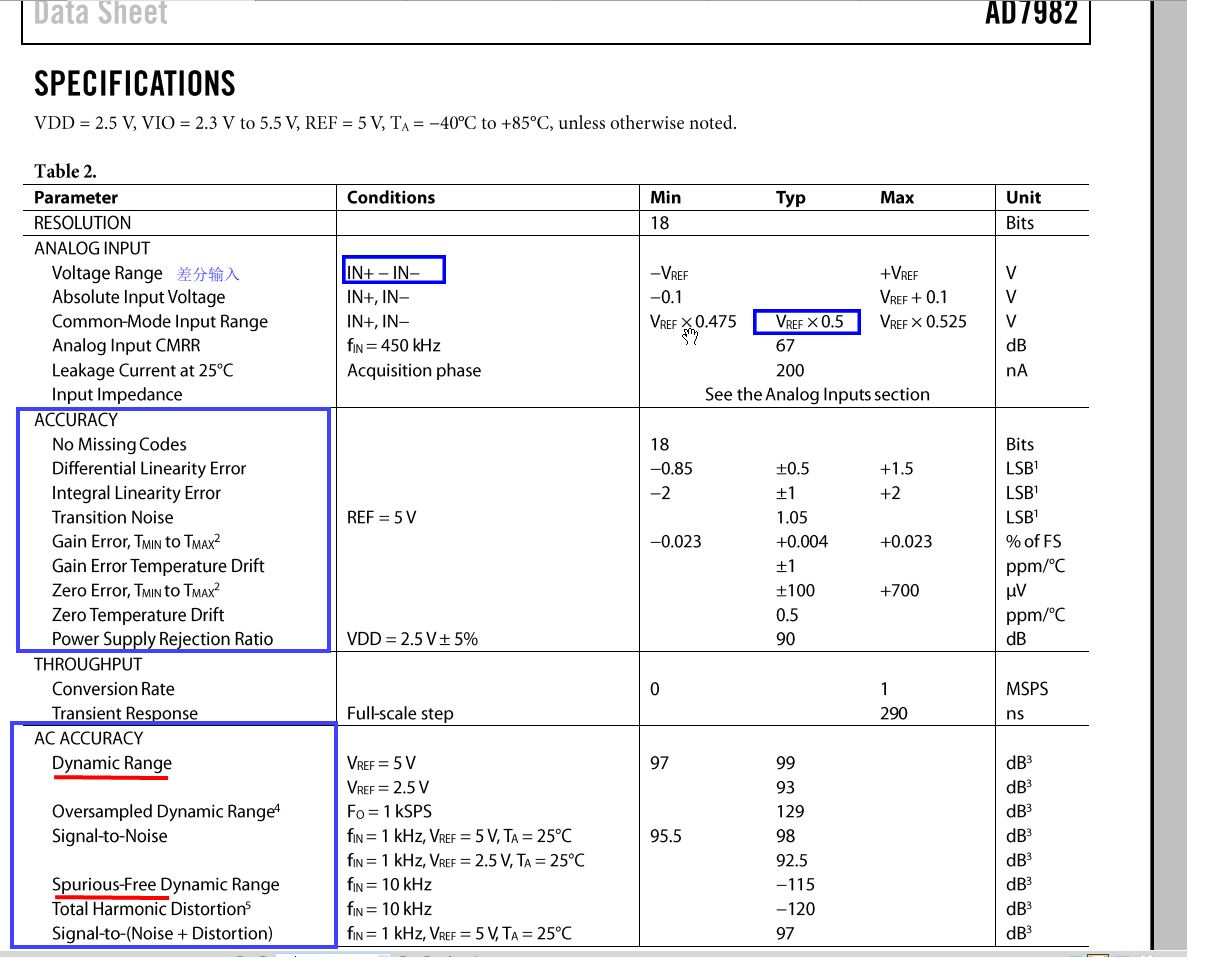If you have a related question, please click the "Ask a related question" button in the top right corner. The newly created question will be automatically linked to this question.

Hi there, we are considering ADS8863 as replace for AD7982 in a cost down project. When comparing AC performance specification I go back to the definitions of SNR, THD,SINAD and SFDR(Spurious free dynamic range).

This referecne article (https://www.ni.com/content/ni/locales/zh-cn/support/documentation/supplemental/06/understanding-frequency-performance-specifications.html#section-1740667349)  gives simple and clear explains on these definitions.

In my understanding, all these specs can be uniformly described as power (or volatge) of Signal divided by X , that is  (Sig/X);  and when represented in decibels, it will be Sig(db) - X(db).  Here the letter X refers to:

(1) rms of noise ,  for SNR

(2) RSS of harmoniscs (9th order for ADS8863) , for THD. (positive dB value)

(3) RSS of rms noise + harmonics, for SINAD.  so SINAD value is always less(worse) than SNR and THD.

(4) max. (worst) spur (harmonically or nonharmonically related spur ) , for SFDR.

My questions are  :

(1)If in a same test setup (as in the ref article, pictures snapshot below), the amplitudes of harmonics and spurs shoud always be higher than the noise floor level (if not , you wont see any "spikes"), so we could intuitively get a Larger SNR dB value than SFDR(or THD) from the definitions. I also marked this idea on the bottom-right figure . However, the actual datasheet data is completely opposite to this thought (Datasheet : SNR 96.5dB < SFDR 112dB) . Something may be wrong with my thinking, hope some expert could advice. This is confusing for me....(2) When choosing or comparing a ADC, how should we use the parameter SFDR ?

Thanks a lot !

• Note:

Found something in MT-003, which says "Note that the theoretical noise floor of the FFT is equal to the theoretical SNR plus the FFT process gain, 10×log(M/2), where M is FFT size." on page1.  This seems could explain why in datasheet SNR is far less than SFDR.

Suppose this is true, one backward question is when I measured an FFT plot on bench test, just like figure 25 in TI slau515a , the actual noise floor can be easily read from this plot, but how should I calculate the actual SNR value with all the bins?I still need more digging to understand what the heck is FFT process gain and how is adds up into SNR. maybe some re-learning on digital signal processing.....

Really appreciate if someone expert could illustrate in a less-mathematical way..thanks very much

• Hello,

I think you are confusing SNR with the FFT noise floor value.  The FFT is made up of multiple frequency bins.  The width of each bin in Hz is simply the sample rate (in Hz) divided by the number of samples.  The frequency bin width is then Fs/N.  If you take more samples to compute the FFT spectrum, the width of each frequency bin will get smaller (Fs stays constant, N increases).  Since the total noise is now spread over more bins, the magnitude of the noise in an individual bin will reduce, but the total noise will not change.  This is what is described as FFT processing gain.

The way you calculate the total SNR from an FFT is the same as calculating THD.  You need to RSS the magnitude of all bins except the bins containing the harmonics and fundamental, and divide this total into the the fundamental value.

Regarding question 1, unless the largest spur is also a harmonic, then it is included in the SNR calculation, which forces the SNR to be less than the SFDR value.  If the largest spur is also a harmonic, then it would be excluded from the SNR calculation.  In this case, if the spur(harmonic) is large enough, you could get a higher SNR value than SFDR.

For question 2, the SFDR is an important metric if you will have a signal that falls at that same frequency.  Even though your noise floor is lower over most of the frequency span, the spur will add additional error at that specific frequency, limiting the overall sensitivity.

How to calculate SINAD from the FFT?  (I am using this as an example since it includes the harmonic FFT bins, but the process is similar for SNR.)

Let's assume we take 8 samples and the FFT magnitude is plotted in dBc, which means your fundamental is 0dBC.  Also, lets assume the fundamental falls in bin 3.  You can then calculate SINAD as follows:

Bn is the bin number (1 to 8 in this example), and the magnitude is dBc.  (which is a negative value)

Regards,
Keith Nicholas

By the way, I got two more questionstoconsult if you have time. Thanks!

(3) I found in TI  ADS8863  datasheet there is only SFDR defined, however ,in ADI  AD7982  there are both DR and SFDR (snapshot as below, in datasheet p3), may I ask how they are different from each other and how to use them respectively ?(4) For ADC acquisition system,  to evaluate SNR we used a method like this before :  From time-domain perspective, we treat the signal conditioning chain and ADC as a whole box,  and took two measurements with all setups identical, except the input signals. In the first measurement we short the input to GND and calculatet the RMS voltage of the "Noise base" of the system, RMS_n. In the second measurement, we use a sine wave input signal and acquire its rms voltage value with ADC output, RMS_sig.  Then simply take 20*log(RMS_sig/RMS_n) as the actual SNR result, which is around 74dB, and the datasheet SNR spec is 77dB. seems close.

How do you think of this "time-domain" method  when comparing with the usually recommended FFT-based frequency-domain method? We would like to hear your insight.

Thanks again !

• Hello,

Dynamic range and SFDR are two very different measurements.  SFDR is simply the largest frequency component that is not DC or the fundamental in the FFT plot.

Dynamic range is closely related to SNR.  It is measured by shorting the inputs, taking several readings and calculating the rms noise.  This noise is then divided into the RMS full scale range of the ADC. For example, if you shorted the inputs of an 18b ADC and measured (in codes LSB) 3LSBrms (or about 18LSB peak to peak), then you could calculate DR as follows:

N=rms noise in LSB's (or codes)

FSR for an 18b ADC is (2^18/2)/(2^0.5)=92682

D.R. = 20*log(FSR/N) = 20*log(92682/3) = 89.8dB

The way your are measuring SNR will get you close, but it will overestimate the actual SNR since your measured full scale range also includes the noise.

Regards,
Keith

• Hi Keith,

Quote:  "The way your are measuring SNR will get you close, but it will overestimate the actual SNR since your measured full scale range also includes the noise.  "

I am not sure I correctly understood your reason on “overestimate the actual SNR”.  Do you mean that  this way also including the noise of the input signal and the noise of upstream signal conditioning circuits?

I agree that signal source and upstream conditioning circuits (like amplifiers) will introduce extra noise in addition to the ADC device's noise, so we are actully measuring the whole signal chain 's SNR_chain (which should be a final goal from the perspective of end-user),  instead of the ADC device SNR_adc.

(a) If we simplify the signal chain as two parts: amplifers and ADC, with respectively SNR_amp and SNR_adc in dBs, will the whole chain SRN_chain = SNR_amp + SNR_adc ?  So you think we get an “overestimated” measured SNR value which is larger than actual ADC SNR.

(b) Another issue is , signal source should be important. Ideally it requires a high-quality sine wave signal source generator with excellent spec on noise, accuracy and THD, to minimize the influence of input signal's noise and distortion . But the real situation is often limited by equipment budget. May I ask how do you deal with this problem in practice ?

Appreciate if you would explain in more details. Thanks!

• Hello,

I probably could have explained this better.  The better way to calculate system SNR is to use the maximum full scale range of your input signal, not the measured value.  If you just want to determine SNR for the ADC, then you would use the full scale input range of the ADC.

Regarding (a), you can do an error analysis (or measurements) for the amplifier section and the ADC separately, and then combine the results for total SNR.  TI Precision Labs - ADC's Noise analysis discusses how to do the math.  You may also want to download the Analog Engineer's Calculator, which includes a noise tool to sum the noise from the input amplifier stage, the reference, and the ADC.  https://www.ti.com/tool/ANALOG-ENGINEER-CALC

(b)  Yes, to measure very high performance ADC's (>18b resolution), you need a very good signal generator, which costs a lot of money.  In order to test with lower performance equipment, you can use a lower cost generator, and then use external bandpass filters at a specific frequency (1kHz, 10kHz, ....) for a lower cost solution.  For 18b ADC's or lower resolution, we have a 2kHz precision generator evaluation board that works very well, but only supports 2kHz with low SNR and good THD.

https://www.ti.com/tool/PSIEVM

Regards,
Keith